CHAPTER 10 REGULAR HOMEWORK I

Resources:

Have you found videos, websites, or explanations that helped you understand this chapter? Let us know and we'll add them to "Resources" part of this page for other students to use.

Question 1

Use the VSEPR model to predict the shape of BeF2.

Select one:ExplanationBe : 2 bonds +  0 lone = linear

Question 2

Use the VSEPR model to predict the shape of SF6.

Select one:ExplanationS: 6 bonds + 0 lone pair= 6 =

Question 3

Use the VSEPR model to predict the shape of SiH4.

Select one:ExplanationSi = 4 bonds + 0 lone pair = 4 =

Question 4

Use the VSEPR model to predict the shape of FCN.

Select one:ExplanationC = 2 bonds =  2 linear

Question 5

Use the VSEPR model to predict the shape of BeF3-.

Select one:Explanation

Be : 3 bonds + 0 lone pairs = 3 = trigonal planar

Question 6

Write the Lewis structure for XeO2 . What is the correct information about the electron-pair arrangement?

Notation AXnEy where A is the central atom, n is the number of X atoms bonded to it, and y is the number of lone pairs of electrons.

Select one:ExplanationXe : 2 bonds + 2 lone pairs = 4 = electron geometry :tetrahedral

Question 7

Write the Lewis structure for I3-. What is the correct information about the electron-pair arrangement?

Select one:ExplanationI : 2 bonds + 3 lone pairs = trigonal bipyramidal

Question 8

Write the Lewis structure for NO2-. What is the correct information about the electron-pair arrangement and the shape of the ion?

Select one:ExplanationN : 2 bonds + 1 lone pair = 3

shape: bent (because the lone pairs' repulsion force is really strong)

Question 9

Write the Lewis structure for PCl5. What is the correct information about the electron-pair arrangement?

Select one:ExplanationP : 5 bonds + 0 lone pair = 5 =  trigonal bipyramidal

Question 10

Write the Lewis structure for AlCl3. What is the correct information about the electron-pair arrangement?

Select one:ExplanationAl : 3 bonds + 0 lone pair = 3  =  trigonal planar.

Question 11

Write the Lewis structure for SeF4. What is the correct information about the electron-pair arrangement and shape of the molecule?

Select one:ExplanationSe : 4 bonds + 1 lone pair = 5 seesaw

Question 12

Write the Lewis structure for SCl2. What is the correct information about the electron-pair arrangement and shape of the molecule?

Select one:ExplanationS : 2 bonds + 2 lone pairs = 4

Molecular Shape: bent

electron geometry: (tetrahedral)

Question 13

Write the Lewis structure for GeH4. What is the correct information about the electron-pair arrangement and shape of the molecule?

Select one:Explanation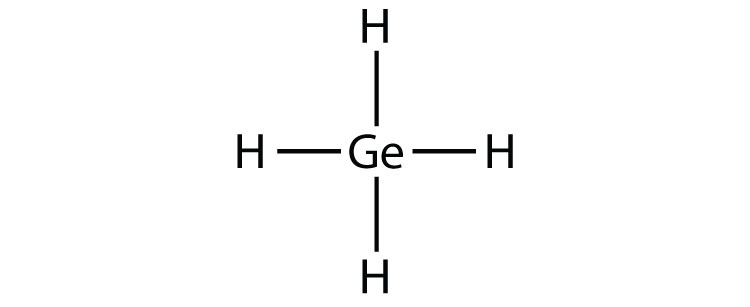Ge: 4 bonds + 0 lone = 4 (tetrahedral)

Question 14

Of the following, which molecule has the smallest bond angle?

Select one:ExplanationH2O has the most amount of lone pairs that causes H2O have the smallest bond angle. Lone pairs cause more repulsion between other elements creating a smaller bond angle.

Question 15

What type of structure does the XeOF2 molecule have? (All the atoms are bonded to the central xenon atom.)

Select one:ExplanationXe : 3 bonds + 2 lone pairs = 5 = T shape

Question 16

In earlier problems in this chapter the shape of the following molecules was found. Use the shape to determine if the molecule is polar or nonpolar. (All must be correct to get credit.)

Select one or more:Explanation

Draw the shapes

BeF2 : linear (equal electronegativity on both sides so nonpolar)

SF6 : equal electron negativity for each exterior elements and symmetrical means nonpolar

FCN : linear but uneven electronegativity so polarQuestion 17

Identify the hybrid orbitals on the central atom that make the bonds in ClF3. First, write the Lewis structure, and give the electron-pair arrangement.

Select one:Explanation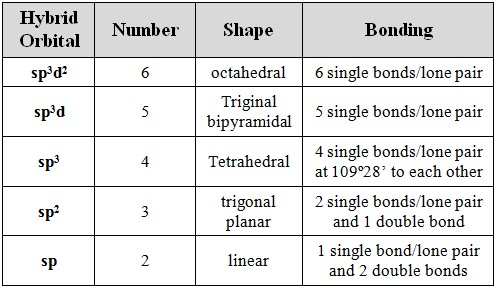Cl : 3 bonds + 2 lone pairs = 5 = trigonal bipyramidal.

Question 18

Identify the hybrid orbitals on the central atom that make the bonds in BBr3. First, write the Lewis structure, and give the electron-pair arrangement.

Select one:Explanation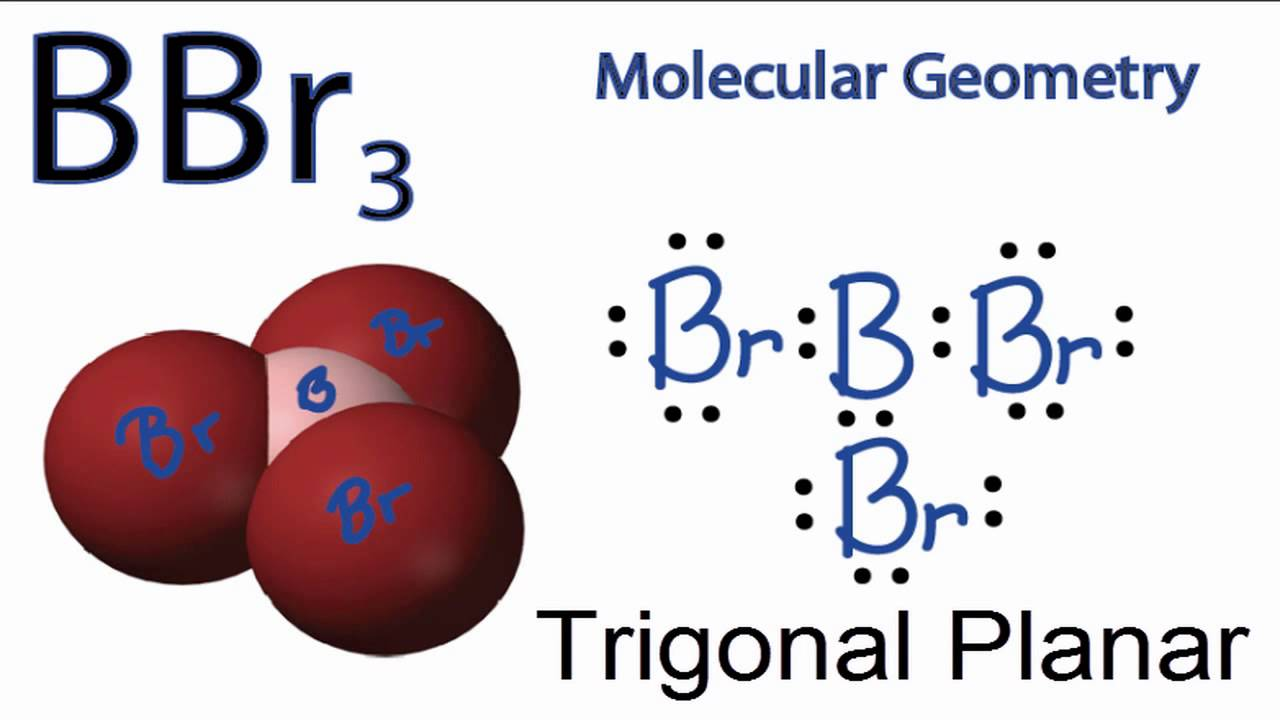Question 19

Identify the hybrid orbitals on the central atom that make the bonds in BeF2. First, write the Lewis structure, and give the electron-pair arrangement.

Select one:Explanation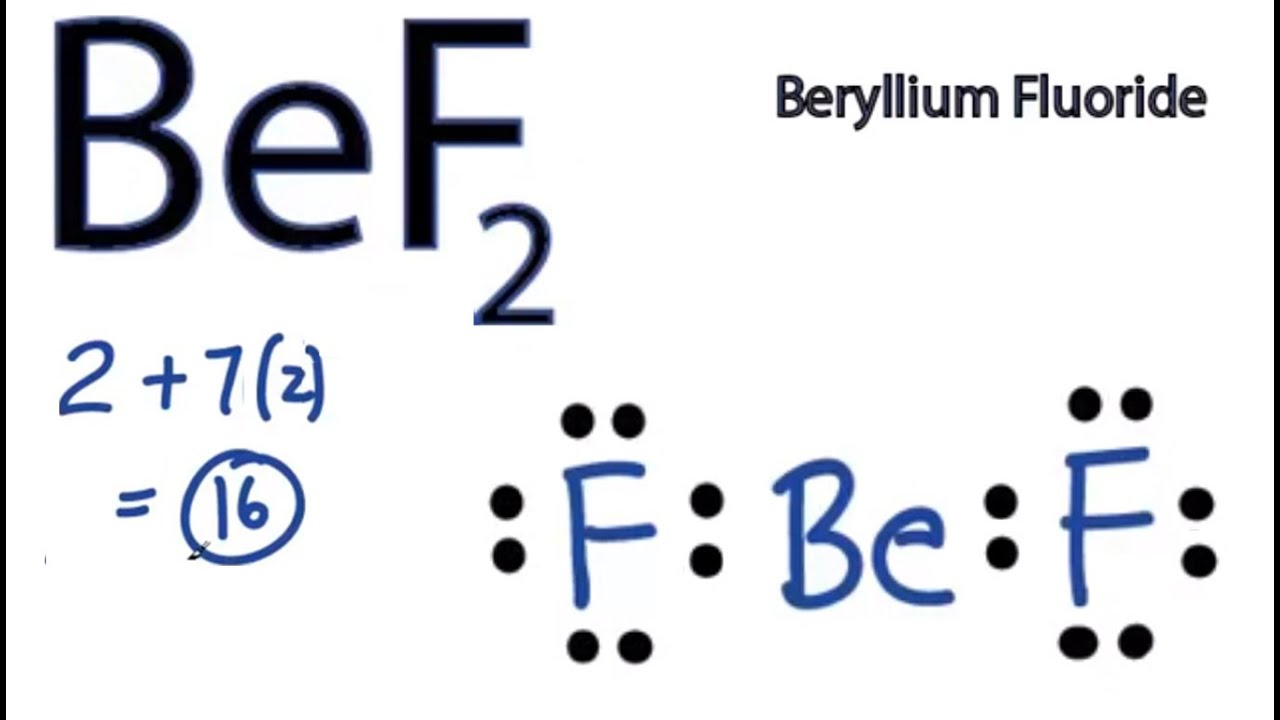Question 20

Identify the hybrid orbitals on the central atom that make the bonds in ONCl. First, write the Lewis structure, and give the electron-pair arrangement.

Select one:ExplanationN : 2 bonds + 1 lone = 3 = trigonal planarQuestion 21

Identify the hybrid orbitals on the central atom that make the bonds in NF3. First, write the Lewis structure, and give the electron-pair arrangement.

Select one:ExplanationN : 3 bonds + 1 lone pair = 4 tetrahedralQuestion 22

Identify the hybrid orbitals on the central atom that make the bonds in H3O+. First, write the Lewis structure, and give the electron-pair arrangement.

Select one:ExplanationO : 3 bonds + 1 lone pair = 4Question 23

Identify the hybrid orbitals on the central atom that make the bonds in SCl4. First, write the Lewis structure, and give the electron-pair arrangement.

Select one:ExplanationS : 4 bonds + 1 lone pair = 5  trigonal bipyramidalQuestion 24

Identify the hybrid orbitals on the central atom that make the bonds in IF5. First, write the Lewis structure, and give the electron-pair arrangement.

Select one:Explanation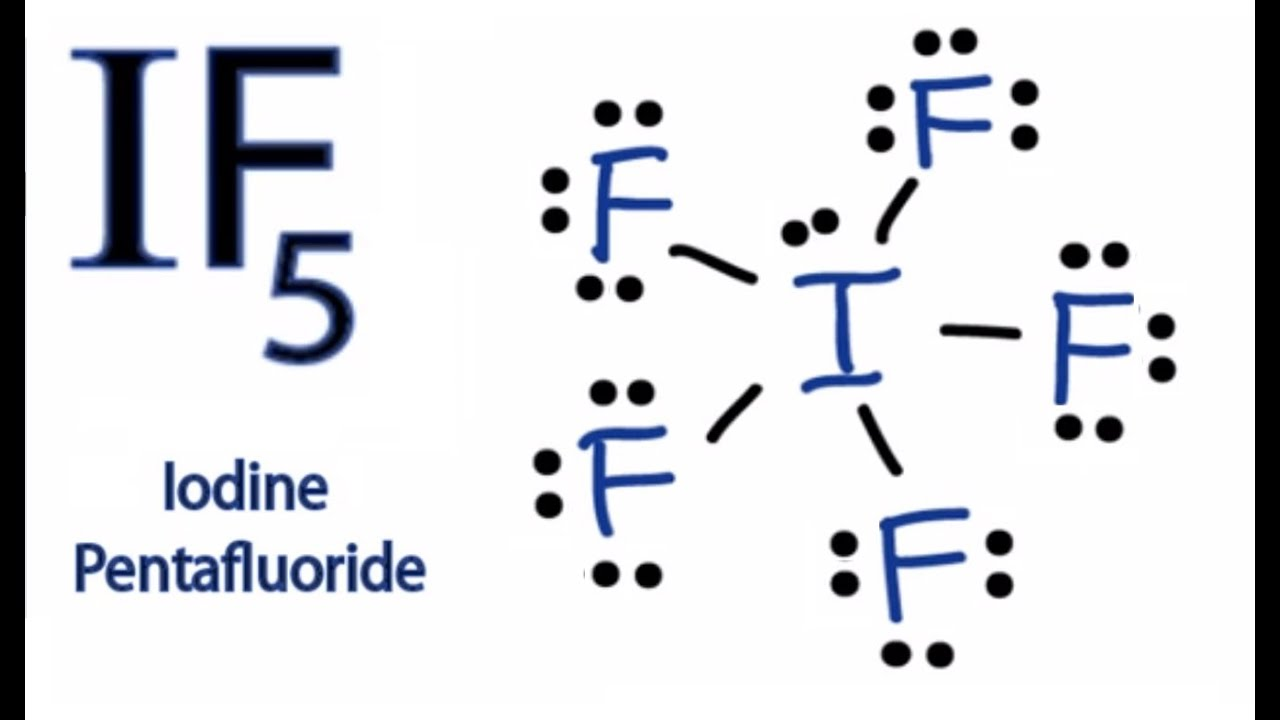I = 5 bonds + 1 lone pair = 6Question 25

Which of the follow bonds is (are) sigma bonds? For each case the z axis is the axis defined by the atoms.

(To help answer this question, draw a picture that shows the overlap of each pair of bonding orbitals. If the electron density of the bonding orbitals is along the axis defined by the atoms, then it is a sigma bond.)

Select one or more:Explanation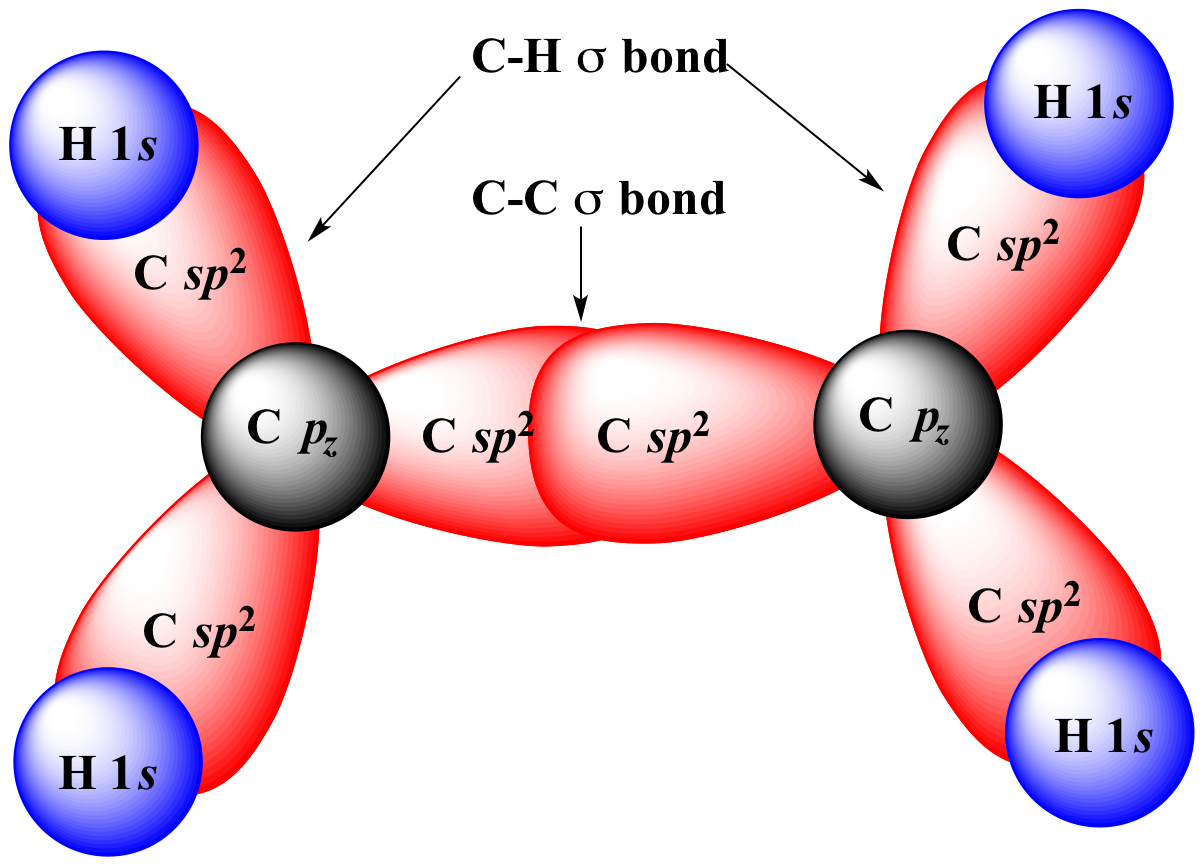Question 26

Draw the Lewis structure of this compound, indicating the F-S-F bond angles and the hybrid orbitals used by sulfur.

Select one:Explanation